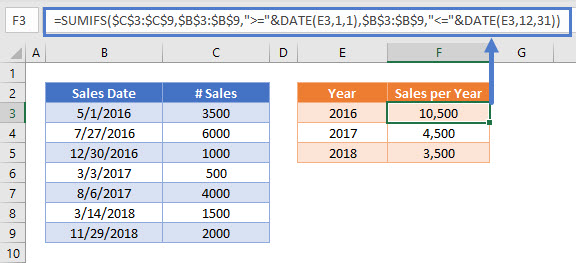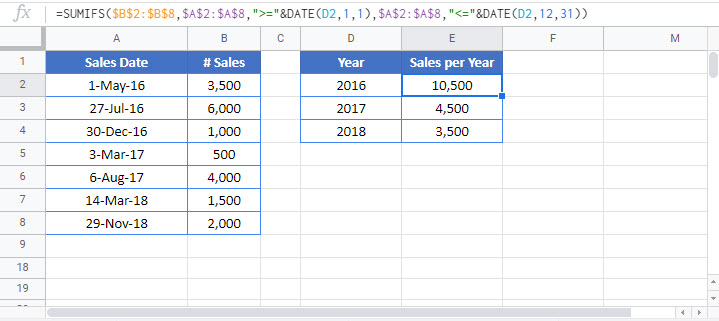# sum if by year- Excel & Google Sheets

This tutorial will demonstrate how to use the SUMIFS Function to sum data with specific years in Excel and Google Sheets.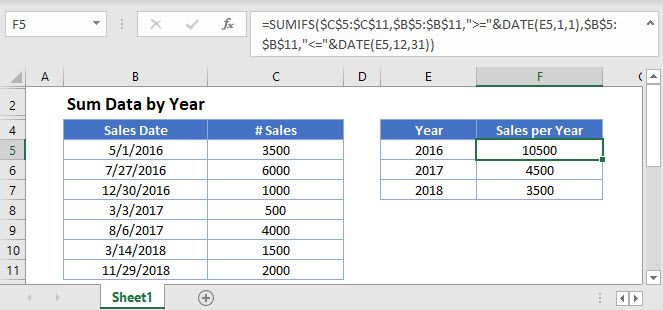## Sum if by Year

The SUMIFS Function sums data corresponding to characteristics you define. This example sums the Number of Sales where the Sales Date is in a specified year.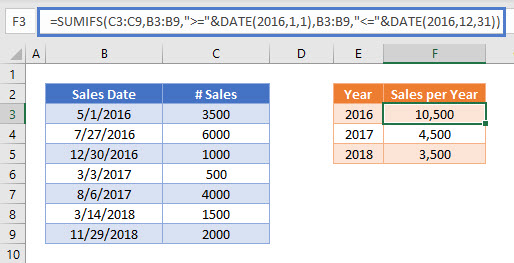You can use the DATE Function to define dates within a SUMIFS Function.

If the year is 2016, the date must be between the first day in January and the last day in December of 2016. The operators in a SUMIFS Function are in double quotes (“”), and they’re joined to the date with an ampersand (&), so the Sales Dates in 2016 are:

• “>=”&DATE(2016,1,1)
• “<=”&DATE(2016,12,31).

## Sum If by Year – Cell References

Usually, it is bad practice to hard-code values into formulas. Instead, it’s more flexible to use a separate cell to define the year instead. As with the DATE Function, join the cell references to the operators with &.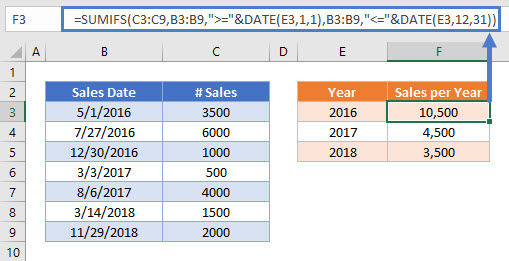## Locking Cell References

To make our formulas easier to read, we’ve shown the formulas without locked cell references:

But these formulas will not work properly when copy-pasted elsewhere within your Excel file. Instead, you should use locked cell references like this: